## Perpendicular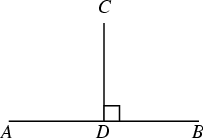Two lines, vectors, planes, etc., are said to be perpendicular if they meet at a Right Angle. In, two Vectors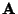andare Perpendicular if their Dot Product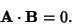In, a Line with Slopeis Perpendicular to a Line with Slope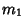. Perpendicular objects are sometimes said to be orthogonal.''

In the above figure, the Line Segmentis perpendicular to the Line Segment. This relationship is commonly denoted with a small Square at the vertex where perpendicular objects meet, as shown above, and is denoted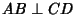.